# How to convert time to decimal number, hours, minutes or seconds in Excel

The tutorial demonstrates different ways to convert time to decimal in Excel. You will find a variety of formulas to change time to hours, minutes or seconds as well as convert text to time and vice versa.

Because Microsoft Excel uses a numeric system to store times, you can easily turn hours, minutes and seconds into numbers that you can use in other calculations.

In general, there are two ways to convert time to decimal in Excel - by changing the cell format and by using arithmetic calculations or Excel time functions, such as HOUR, MINUTE and SECOND. Further on in this tutorial, you will find the detailed explanation of the first way and formula examples demonstrating the other technique.

## How to convert time to decimal number in Excel

Overall, there are three ways to change a time value to a decimal number: arithmetic operation, CONVERT function or a combination of three different Time functions.

The easiest way to convert time to decimal in Excel is to multiply the original time value by the number of hours, seconds or minutes in a day:

• To convert time to a number of hours, multiply the time by 24, which is the number of hours in a day.
• To convert time to minutes, multiply the time by 1440, which is the number of minutes in a day (24*60).
• To convert time to seconds, multiply the time time by 86400, which is the number of seconds in a day (24*60*60 ).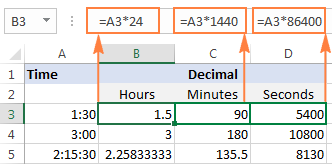In the following sections, you will learn the other methods of converting times to a decimal number in Excel.

### How to convert time to hours in Excel

This section demonstrates 3 different formulas to convert hours from the standard time format (hh:mm:ss) to a decimal number.

#### Formula 1: Arithmetic calculation

You already know the fastest way to convert a time value to a number of hours in Excel - multiplying by 24, i.e. by the number of hours in one day:

`=A2*24` (where A2 is the time value)

To get the number of compete hours, embed the above formula in the INT function that will get rid of the fractional part:

`=INT(A2*24)`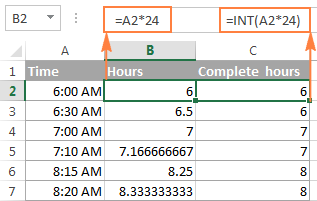#### Formula 2: CONVERT function

Another way to perform the "time > hours" conversion is to use the following Convert formula:

`=CONVERT(A2, "day", "hr")`

#### Formula 3: HOUR, MINUTE and SECOND functions

Finally, you can use a bit more complex formula, whose logic, however, is quite obvious. Extract the individual time units by using the HOUR, MINUTE and SECOND functions, then divide minutes by 60 (the number of minutes in an hour) and seconds by 3600 (the number of seconds in an hour), and add up the results:

`=HOUR(A2) + MINUTE(A2)/60 + SECOND(A2)/3600`

### How to convert time to minutes in Excel

The same three methods can be used to convert minutes from the standard time format to a decimal number.

#### Formula 1: Arithmetic calculation

To convert time to total minutes, you multiply time by 1440, which is the number of minutes in one day (24 hours * 60 minutes = 1440):

`=A2*1440`

If you want to return the number of compete minutes, utilize the INT function like in the previous example:

`=INT(A2*1440)`

You can view the results in the screenshot below: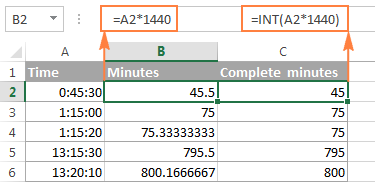#### Formula 2: CONVERT function

To do the "time > minutes" conversion with the CONVERT(number, from_unit, to_unit) function, supply "day" as the unit to convert from and "mn" as the unit to convert to:

`=CONVERT(A2, "day", "mn")`

#### Formula 3: HOUR, MINUTE and SECOND functions

One more way to get the number of minutes is to multiply hours by 60 and divide seconds by the same number:

`=HOUR(A2)*60 + MINUTE(A2) + SECOND(A2)/60`

### How to convert time to seconds in Excel

Converting time to total seconds in Excel can be done in a similar fashion.

#### Formula 1: Arithmetic calculation

Multiply the time value by 86400, which is the number of seconds in a day (24 hours * 60 minutes * 60 seconds = 86400):

`=A2*86400`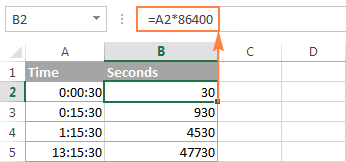#### Formula 2: CONVERT function

The formula is basically the same as in the above examples with the only difference that you convert the "day" unit to "sec":

`=CONVERT(A2, "day", "sec")`

#### Formula 3: HOUR, MINUTE and SECOND functions

Use the HOUR, MINUTE and SECOND functions like in the two previous examples (I believe at this point you don't need any further explanation of the formula's logic :)

`=HOUR(A2)*3600 + MINUTE(A2)*60 + SECOND(A2)`

Tips:

1. If any of the above formulas returns a value formatted as time, simply change the cell's format to Generalto display it as a number.
2. To convert time to a decimal number that represents the time in the internal Excel system, apply the General format to the cell. With this approach, 23:59:59 will be converted to 0.99999, 06:00 AM to 0.25, and 12:00 PM to 0.5. If an integer part of the converted number is greater than zero, it means that your cell contains both date and time values.

## How to split date and time in Excel

As is often the case, your Excel worksheet may contain dates and times in one cell, while you want to split them into two separate cells.

Remembering that in the internal Excel system the date value is stored as a whole part and the time value as a fractional part of a decimal number, you can extract the date using the INT function, which rounds the cell value down to the nearest integer.

Supposing your original dates and times are in column A, the following formula will accomplish the separation:

`=INT(A2)`To extract the time portion, subtract the date returned by the above formula from the original date and time value:

`=A2-B2`

Where column A contains the original date & time values and column B contains the dates returned by the INT function.

If you'd rather not have time values linked to the separated dates (for example, you may want to remove the date column in the future), you can use the following MOD formula that refers to the original data only:

`=MOD(A2,1)`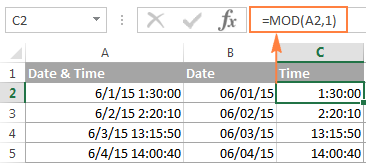Tip. If the separated date and time values are not displayed properly, change the format of the new columns to Date and Time, respectively.

This is how you split date and time in Excel. If you want to further separate hours, minutes and seconds into individual columns, then use the HOUR, MINUTE and SECOND functions, as demonstrated in How to get hours, minutes and seconds from a timestamp.

## How to spell time in Excel

Sometimes, you may need to convert time into the format that reads something like "# days, # hours, # minutes and # seconds". A good thing is that you already know all the ingredients of the formula:

• Extract days using the INT function;
• Extract time units with HOUR, MINUTE and SECOND functions, and
• Concatenate all parts in a single formula.

Having difficulties with figuring out a proper formula for your worksheet? The following example will make things easy!

Supposing you have the dates of upcoming events in column B beginning in cell B4, and the current date and time returned by the NOW() function in cell B1.

The formula to calculate the time difference is as simple as `=B4-\$B\$1`. Of course, nothing prevents you from subtracting the current date and time directly with `=B4-NOW()`.

And now, let's make a countdown timer that would show how many days, hours, minutes and seconds are left until each event.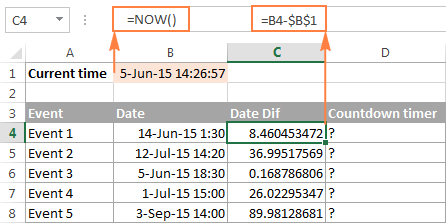The formula to enter in cell D4 is as follows:

`=INT(C4) & " days, " & HOUR(C4) & " hours, " & MINUTE(C4) & " minutes and " & SECOND(C4) & " seconds"`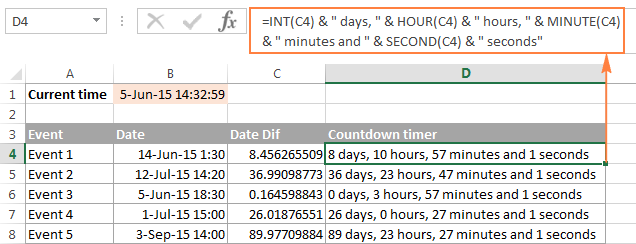If you wish to get rid of 0 values, like in cells D6 and D7 in the screenshot above, then include the following IF statements:

`=IF(INT(C4)>0, INT(C4)&" days, ","") & IF(HOUR(C4)>0, HOUR(C4) & " hours, ","") & IF(MINUTE(C4)>0, MINUTE(C4) & " minutes and ","") & IF(SECOND(C4)>0, SECOND(C4) & " seconds","")`

All zeros are gone!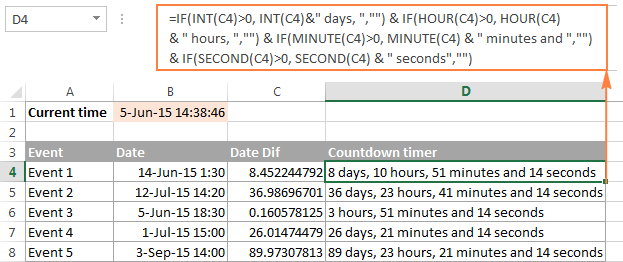Note. When either of the above formulas refers to a negative number, the #NUM! error will appear. This may happen when you subtract a bigger time from a smaller one.

An alternative way to write time in words in Excel is to apply the following custom time format to the cell: d "day," h "hours," m "minutes and" s "seconds". No formulas and no calculations are required! For more information, please see Creating a custom time format in Excel.

## Convert text to time in Excel

If your time formulas and calculations do not work right, time values formatted as text is often the cause. The fastest way to convert text to time in Excel is using the TIMEVALUE function.

The Excel TIMEVALUE function has just one argument:

TIMEVALUE(time_text)

Time_text is a text string in any of the time formats that Excel can recognize. For example:

`=TIMEVALUE("6:20 PM")`

`=TIMEVALUE("6-Jan-2015 6:20 PM")`

`=TIMEVALUE(A2)` where A2 contains a text string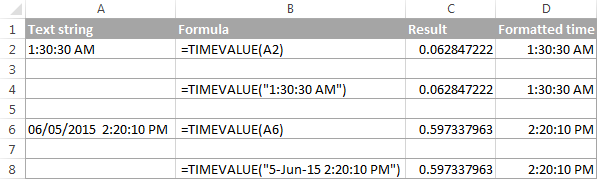As you see, the formulas with cell references and corresponding text strings deliver identical results. Also, please notice the left alignment of time strings (text values) in cells A2 and A6 and right-aligned converted time values in column D.

## Convert time to text in Excel

Supposing you have an Excel file full of times formatted to look like "8:30:00 AM" and you want to convert them to the text format. Simply changing the cell's format to TEXT won't work because this would change your time values to underlying numeric representation of the time. For example, 8:30:00 AM will be converted to decimal 0.354166666666667.

So, how do you convert cells to the text format so that your cells still have the time in them? The answer is to use the TEXT function that converts a numeric value to text with the display formatting that you specify, for example:

`=TEXT(\$A2,"h:mm:ss")`

The screenshot below demonstrates other possible formats: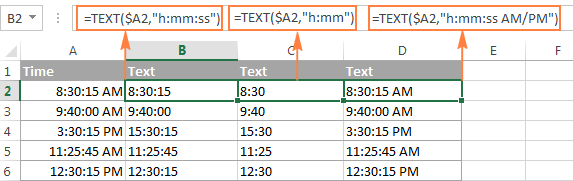## How to convert numbers to time format in Excel

If you have a list of numbers such as 1, 2, 3.5 and you want to convert them to a time format, for example 1:00:00, 2:00:00 or 3:30 AM, perform the following steps.

1. Divide the numbers by 24 (there are 24 hours in a day). The formula can be as simple as =A2/24.
2. Select the cell(s) with the formula result, right-click and select Format Cells from the context menu or press Ctrl+1. Either way, the Format Cells dialog will appear, you select Time on the left pane under Category, and choose the format you want on the right pane under Type. Please see How to apply or change the time format in Excel for more details.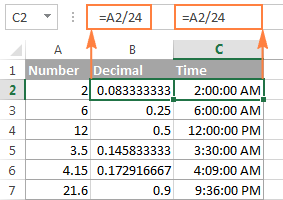That's all for today. If someone wants to get the first-hand experience with the formulas discussed in this article, you are most welcome to download the Sample workbook for converting time in Excel.

If you want to learn a few more helpful formulas to add and subtract times, calculate elapsed time and time difference, please check out other parts of our Excel Times tutorial (the links are available at the end of this page). I thank you for reading and hope to see you again next week.

### 352 comments to "How to convert time to decimal number, hours, minutes or seconds in Excel"

1.Sean says:

I have the following in a spread sheet and am wondering how I go about setting up a formula to learn the difference between the cells. It is a HUGE data sheet with almost 20k entries. The day as just a number and not a full date is throwing me off.

Day Hour:Min:Sec

29 18:25:26 29 18:39:12

•Sean says:

The data points should have looked like this
29 18:25:26
29 18:39:12

2.Addam Accettella says:

How do I go from 0800-1200 to 8:00am-12:00pm In One cell

3.Khuch Khana says:

I always search here when I stuck in any excel issue and I have never been disappointed. Keep up the good work.

4.JZ says:

Hi, I have a dataset with time being in the General number format. I know I can manually select the cell to be formatted in time, but don't want to do it manually for all the rows. Can someone tell me how the conversion works? Thanks

For example:
44653.8395833333 is equivalent to 4/2/2022 8:09:00 PM

5.Alyssa says:

Hello, I have an excel sheet with the time in format "11 Days 2 Hours 58 Min 0 Sec" I want to convert this into either a numeric format or just into minutes. What formula could I use to accomplish this?

6.Roger says:

Good morning. I am trying to count the presence of specific digits *within* a column of time values. For example, how many minutes in a column of times end in the character 0? I can't find that anywhere online, and experimenting with COUNT, COUNTIF and COUNTIFS gives me obviously bad answers. Please help?

•Alexander Trifuntov (Ablebits Team) says:

Hello!
Convert a time value to text with the TEXT function. Then extract the last digit using the RIGHT function and count matches with "0".
The formula below will do the trick for you:

=SUM(--(RIGHT(TEXT(A1:A10,"hh:mm"),1)="0"))

•Roger says:

Thank you. I think we're a lot closer to success, but I'm getting a #VALUE! error with that.

I used TEXT function to convert the time column to a column of pure text that looks like it should, basically 1746 rows of values like this...

18:01
18:48
20:15
20:45
21:35
21:59
22:10
22:30
22:59
23:32
05:30

That worked. Then I pasted your formula, modified for all the row numbers in column E containing the data...

=SUM(--(RIGHT(TEXT(e3:e1748,"hh:mm"),1)="0"))

...and got the #VALUE! error. If you can help, I'd hugely appreciate!

•Alexander Trifuntov (Ablebits Team) says:

Hi!
Unfortunately, I was unable to reproduce your issue. The formula is designed to work with time values, but it can also work with times written as text. With the data you provided, it also works correctly.

7.Arnold Nemeth-Zseli says:

Dear Sir,
I have to compare two time sets and check which of them appeared earlier.
so using the delta between TIME A and TIME B:
DELTA = TIME A - TIME B

if Delta is >0, than no problem, i will get the time difference and can be transformed to Text, etc. in order draw an average.
BUt, if Delta is <0, i have a problem because (TIME*24) is not working if the Time is a negative number.

I hope my explanation is clear.
regards,

8.Natalie Williams-Frye says:

If I need to have the clock in times and clock out times to be converted to decimal values that consist of 0-4 minutes = 0 , 4-9 =.1, minutes, can the formula be set up to do the conversions for me?

•Alexander Trifuntov (Ablebits Team) says:

Hi!
Have you tried the ways described in this blog post? If they don’t work for you, then please describe your task in detail, I’ll try to suggest a solution.

9.Victoria says:

Hello,

I was wondering how to translate a time written as a whole number to a time value. For example, the values I have on my spreadsheet are values listed as "543" for 5:43 AM and "1350" for 1:50 PM. I also have values that are "50" for 12:50 AM. Can you please help me translate these values into a time value?

Thank you

•Alexander Trifuntov (Ablebits Team) says:

Hello!
To create a time value, use the TIME function.

=TIME(IF(LEN(A1)=2,0,LEFT(A1,LEN(A1)-2)),RIGHT(A1,2),0)

10.Jamie says:

Hello,

I am struggling with something that might be simple so I apologize in advance if it is.

I'll give an example:
it takes 00h 00min 03sec to bake a cookie
I want to know how much time it will take to bake 500k cookies.
so I want excel to multiply 00h 00min 03sec with 500k
The result should be same format 00h 00min 03sec. I also need to be able to convert the hours in days if needed.

Jamie

•Alexander Trifuntov (Ablebits Team) says:

Hi!
If I understand your task correctly, try the following custom time format:

"0h 0min" [ss]"sec"

Hope this is what you need.

11.Filips says:

Hello, I am working as a logistics coordinator and i have 2 questions:

1) If i have a driver who finishes driving at 18:00(F7) and starts next day at 06:00(E8) it means he has 12hrs of rest time. I used formula =24-F7+E8. But if on sunday he doesnt drive at all. For example finishes saturday at 14:00 but starts only monday at 06:00. What formula could i use to automatically add 24 if there is a blank cell?

2) Is it possible to use format not ''12:45'' but ''12h 45min'' when it counts the rest time?

Thank you

12.Iftkhar Ahmad says:

10 Day(s) 22 Hr(s) 34 Min(s)

How to convert this into days.

•Alexander Trifuntov (Ablebits Team) says:

Hi!
To convert hours to days, divide by 24. To convert minutes to days, divide by 1440.

•Iftkhar Ahmad says:

Hi Sir , Thanks for the response.

i have tried that the same but not getting the exact days.

10 Day(s) 22 Hr(s) 34 Min(s) - I want to convert this in days only , like 10days 22 hrs should come as 11 days. seeking for your help on this.
Thank You